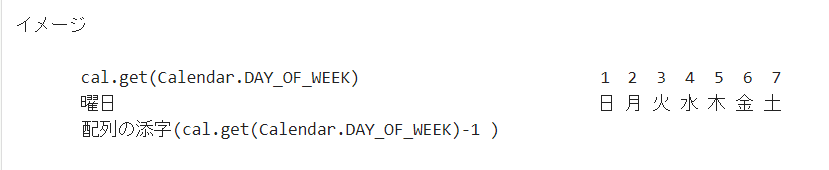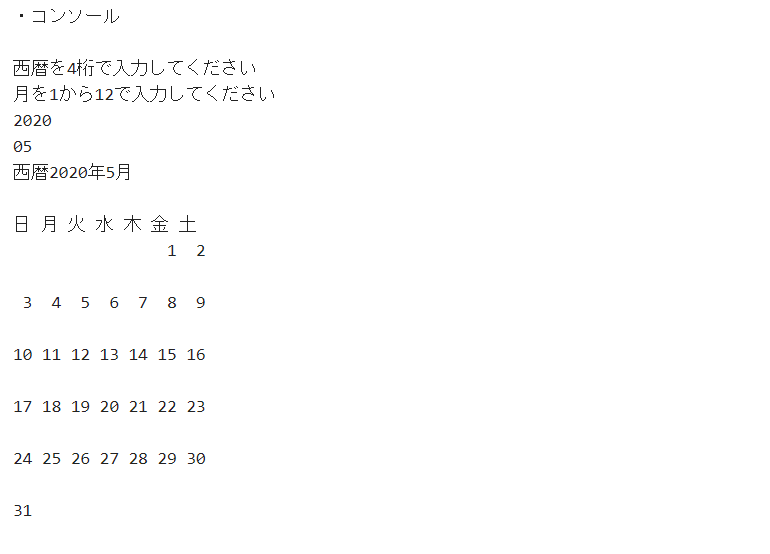# I tried to display the calendar on the Eclipse console using Java.

Just for notes. ・ When cal.set (int year, int month-1,1), it was easy to forget that the month was set from "0 to 11" ⇒ "January to December". -You can find out how many days there are in xx month of xxxx by cal.getActualMaximum (Calendar.DATE). -The days of the week and dates are stored using an array.・ A class that creates and outputs a calendar 　 package foo; import java.util.Calendar; public class Calmaker {

``````public void Makecal(int year,int month){
Calendar cal = Calendar.getInstance();
//int weekday = cal.get(Calendar.DAY_OF_WEEK);
//System.out.println(weekday);

int days[][] = new int;
``````

System.out.println ("Sun Mon Tue Wed Thu Fri Sat"); int row = 0;

//一日から最終日までループ for (int i = 1; i <= cal.getActualMaximum(Calendar.DATE); i++) { //日付けをオブジェクトに設定 cal.clear(); cal.set (year, month-1, i); // Since the month is specified from 0 to 11, I will give it -1. int weekday = cal.get(Calendar.DAY_OF_WEEK);

//日付を拡張 days[row][weekday-1] = i ; //System.out.println(days[row][weekday-1]);

``````	if(weekday % 7 ==0) {
row ++;
}
}
``````

//出力 for (int k = 0; k < 6; k++) { for (int j = 0; j < 7; j++) {

``````			String res = String.valueOf(days[k][j]);

if(days[k][j]==0) {
System.out.print("   ");
}else if(days[k][j] < 10) {
System.out.print(" " + res + " ");

}else if(days[k][j] >= 10) {
res = res+" ";
System.out.print(res);
}
if(j == 6) {
System.out.println("\r\n");
}
}
}
}
``````

} ・ Main class

package foo;

import java.util.Scanner;

public class Makecal {

``````public static void main(String[] args) {
``````

// TODO auto-generated method stub System.out.println ("Enter the year in 4 digits"); Scanner sc1 = new Scanner(System.in); System.out.println ("Enter month from 1 to 12"); Scanner sc2 = new Scanner(System.in);

``````	int year  = sc1.nextInt();
int month = sc2.nextInt();
``````

System.out.println ("year" + year + "year" + month + "month"); System.out.println("");

``````	Calmaker clm = new Calmaker();
clm.Makecal(year,month);
}
``````

}the end.